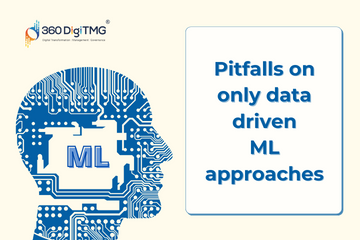Home / Blog / MLOps / KNN Classifier

# KNN Classifier

• July 17, 2023
• 5229
• 39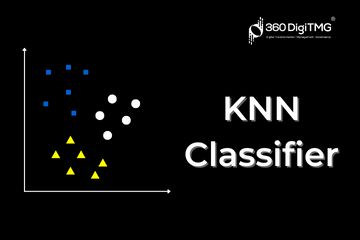### Meet the Author : Mr. Bharani Kumar

Bharani Kumar Depuru is a well known IT personality from Hyderabad. He is the Founder and Director of Innodatatics Pvt Ltd and 360DigiTMG. Bharani Kumar is an IIT and ISB alumni with more than 17 years of experience, he held prominent positions in the IT elites like HSBC, ITC Infotech, Infosys, and Deloitte. He is a prevalent IT consultant specializing in Industrial Revolution 4.0 implementation, Data Analytics practice setup, Artificial Intelligence, Big Data Analytics, Industrial IoT, Business Intelligence and Business Management. Bharani Kumar is also the chief trainer at 360DigiTMG with more than Ten years of experience and has been making the IT transition journey easy for his students. 360DigiTMG is at the forefront of delivering quality education, thereby bridging the gap between academia and industry.

### Introduction

The K-Nearest Neighbours (KNN) algorithm belongs to the group of algorithms for supervised machine learning. Although it may be used to predict numeric data (regression), it is mostly used to predict non-numeric classes (classification). As a result, we have models for KNN regressor prediction as well as KNN classifiers. But the KNN classification method is quite popular in the sector. Since it just memorises the training data and does not generate a discriminative function from the data, it is most commonly referred to as the lazy learner algorithm. Since there is no training phase, it does not concentrate on developing the model but rather constantly looking at the closest data points to classify the data. The KNN model is frequently referred to as a non-parametric algorithm since it makes no assumptions about the data.

### Theoretical Steps for implementing KNN Algorithm:

K-Nearest Neighbors (KNN) algorithm predicts a class of a new data point by using feature selection where the class of the new data point is determined by how closely is it matching with the other data points in the training data. We can theoretically comprehend the working of KNN using the following steps:

Step 1: To implement any algorithm, we need to first load the data which includes the training and the test data. Also, we need to install and import the modules/packages as per the requirement.

Step 2: Exploratory Data Analysis (EDA) techniques are necessary to comprehend the descriptive statistics of our data. Using these techniques, we can locate the central tendencies (mean, median, and mode), characterise the data's distribution (variance, standard deviation, and range), and assess the direction (skewness) and degree (kurtosis) of spread. Depending on the dataset and the business purpose, we may also undertake univariate analysis (histogram or boxplot), bivariate analysis (scatter plot), or multivariate analysis (pairs plot).Step 3: Data Cleaning is a mandatory step because unclean data gives inaccurate results. We can clean the data using the following steps based on the necessity of your dataset:

3.1 Typecasting: Converting one datatype to another datatype (floating-point to integer, array to list, etc.)

3.2 Duplicates: If the value in every cell of 2 rows is the same, we can consider them duplicate values and eradicate them.

3.3 Outlier Treatment: The outliers are frequently shown visually using boxplots. To deal with outliers, we can employ the 3R approach (Rectify, Retain, or Remove). Correcting the values at the source of collection is desirable. If the data is reliable, we may employ the retention strategy and either winsorize the data or round off the outliers to the lowest and maximum values. Trimming can be used to eliminate the outliers; however it is not advised because it results in data loss.3.4 Zero or Near Zero Variance: We ignore the columns which have the same entry in every cell. For example: If the name of the country is India for every person in our dataset, then we cannot analyze the performance based on the country. Hence, we can ignore that feature. Only if there is a variance in the dataset, there will be scope for analysis. Thereby, zero and near-zero variance features are ignored.

3.5 Discretization/Binning: We may transform our continuous data into discrete bins for a clearer visualisation and comprehension of the data as continuous data is usually challenging to visualise given the infinite potential values and minute decimals levels.3.6 Missing Value Handling: As we cannot build an explicit model with missing values, we can consider imputation methods where the numeric values can be replaced using mean/median imputation and non-numeric values can be replaced using mode imputation.

3.7 Dummy Variable Creation: It is preferable to convert non-numeric data to numeric data using various dummy variable creation methods, such as Label encoding for converting Ordinal Data to Interval Scale and One Hot Encoding for converting Nominal Data to Binary Case, which aids in model building, since it is impossible to build a function using non-numeric data considering the computations involved.

3.8 Standardization and Normalization: These methods are used to address the scale issues and make our data scale-free and unitless. Standardization is used to alter the distribution of data by mapping all the values to a common Z-scale where we make the mean=0 and standard deviation=1. Normalization is applied to alter the magnitude of data and bring all the data points to the range of [0,1] so that numerical advantage is not given to any column due to high or low magnitude. In case of inconsistency in data, we can apply various transformation methods until the data becomes consistent which can be visualized using the Normal Quantile-Quantile plot.

Step 4: The optimal value for k, which represents the number of closest neighbours, must be selected once our data has been preprocessed and is prepared for the KNN model construction in order to provide a correct fit model with excellent accuracy and minimal error.

Step 5: Depending on the value of k, we need to calculate the distance between the data points and our new data point. The distance can be calculated using Euclidean (most preferred), Manhattan, or Hamming distance metrics.

In the case of a 2 class problem, we can consider the following cases to classify our new data point:

Case 1: If k (number of nearest neighbors) = 1: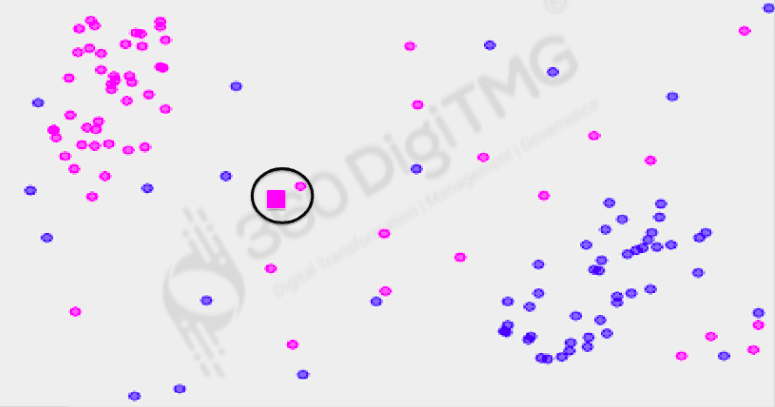Here, since we want to consider only one nearest neighbor(k=1), the class of the data point with the closest distance will be assigned to our new data point.

Case 2: If k (number of nearest neighbors) = 2:The class of the data point with the shortest distance between the two closest data points will be allocated to our new data point because we are only interested in the two nearest neighbours in this case (k=2). It would be challenging to anticipate the class of the data points if the two data points were equally spaced apart from the new data point since this is a two-class problem. Therefore, it is advisable to refrain from selecting the number of nearest neighbors(k) as n or multiples of n for n-class situations.

Case 3: If k (number of nearest neighbors) is 3 or greater than 3(k>=3):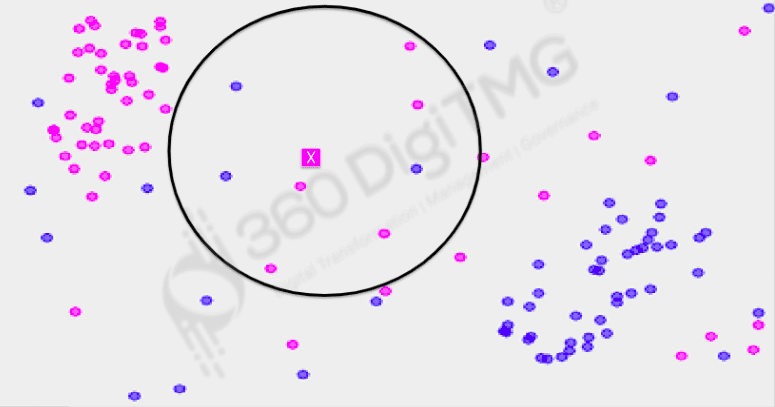If we want to consider three or greater than three nearest neighbors(k>=3), the class of the new data point will be the class of majority of the data points. Since here we have chosen k=8 and majority of the data points with the closest distance to the new data point are of pink class and not purple class, the new data point also will be assigned to the pink class.

Step 6: Analysis of the Misclassified Records: Once, the model has been built, we can analyse the accuracy and error using a contingency matrix which speaks about the correct and incorrect predictions.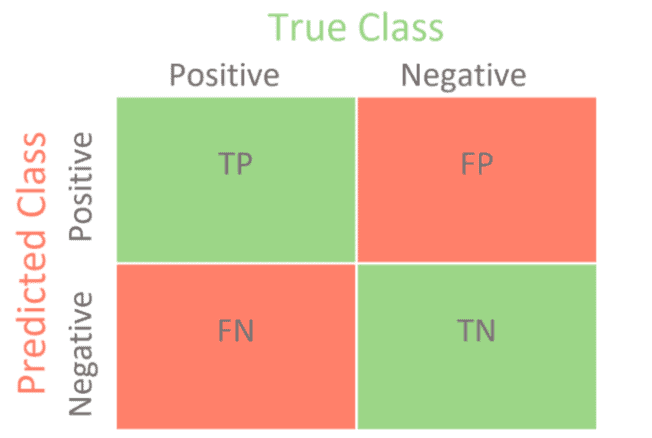Image Source: towardsdatascience

TP: True Positives: This metric indicates the number of instances where positive data was correctly predicted as positive.

FP: False Positives: This metric indicates the number of instances where negative data was incorrectly predicted as positive.

FN: False Negatives: This metric indicates the number of instances where positive data was incorrectly predicted as negative.

TN: True Negatives: This metric indicates the number of instances where negative data was correctly predicted as negative.

Accuracy: This metric is calculated by measuring the proportion of the correctly predicted values in the total number of predictions.

Accuracy=(TP+TN)/(TP+TN+FP+FN)

Error: This metric is calculated by measuring the proportion of the incorrectly predicted values in the total number of predictions.

Accuracy=(FP+FN)/(TP+TN+FP+FN)

Precision: This metric is used for identifying the proportion of True Positives in the total number of positive predictions.

Precision=TP/(TP+FP)

Sensitivity (Recall or Hit Rate or True Positive Rate): This metric is used for identifying the proportion of True Positives in the total number of actually positive instances. Sensitivity=TP/(TP+FN)

Specificity (True Negative Rate): This metric is used for identifying the proportion of True Negatives in the total number of negative predictions.

Specificity=TN/(TN+FP)

Alpha or Type I error (False Positive Rate): This metric is applied for identifying the incorrectly predicted False Positive values in the total number of negative predictions.

α=1-Specificity

F1 Score: F1 rate indicates the harmonic mean and balance between precision and recall. It can assume values between 0 to 1 which indicate the level of balance maintained between precision and recall.
F1 Score=2 x ((Precision x Recall)/(Precision + Recall))

### Implementation of KNN using Python: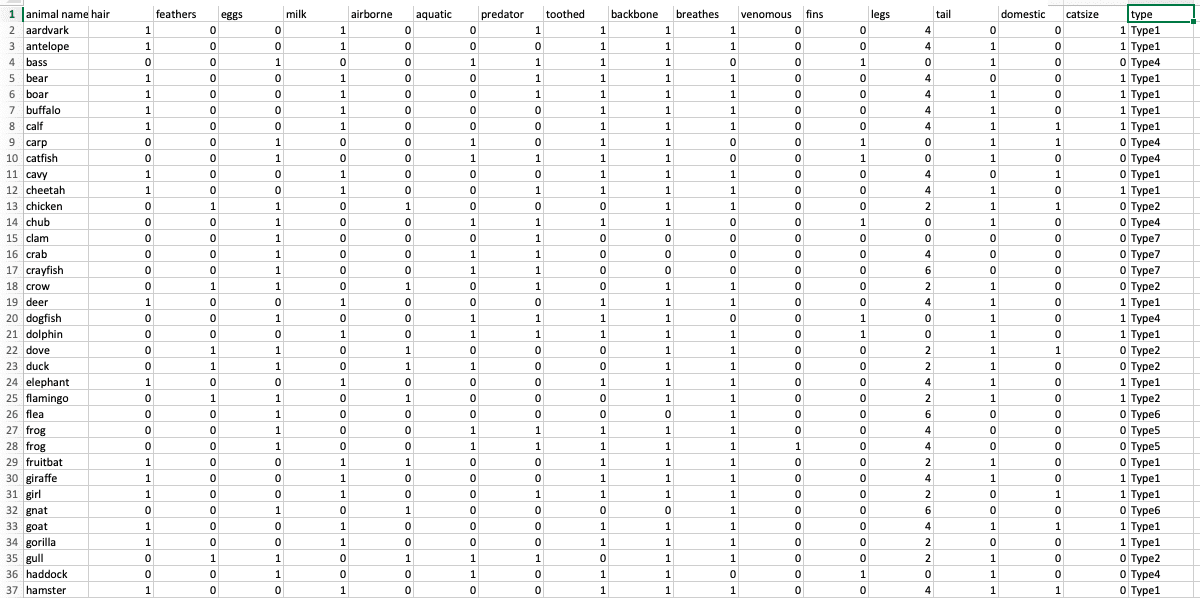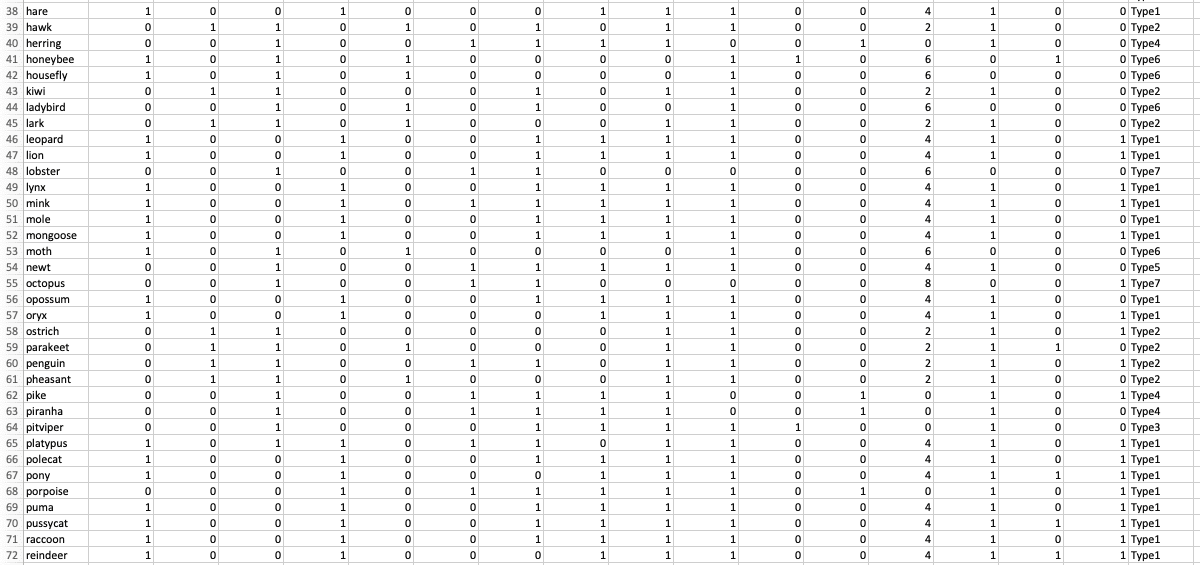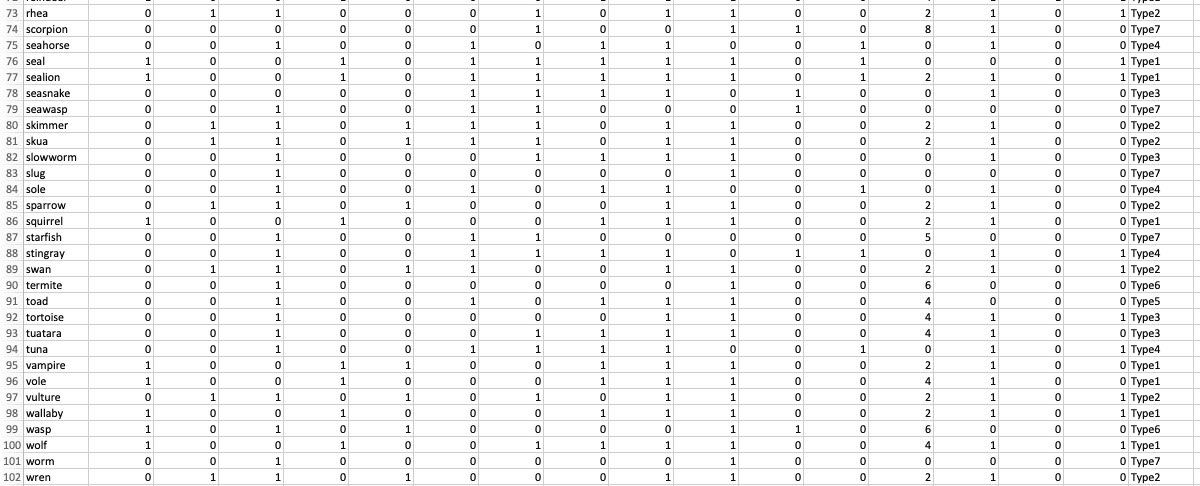We can observe that there are 102 rows and 18 columns which are describing the characteristics of various animals. The objective of the project is to determine the ‘type’ of animal-based on the features. Since we are having historical data with a labeled dataset (‘type’ column), we can use a Supervised Machine Learning algorithm. Since our target is non-numeric data, we can use any of the classification techniques. Here, we are going to implement K-Nearest Neighbors (KNN) classifier on our dataset.

### Steps for Implementing KNN in Python:

Step 1: Load the dataset and import the required modules.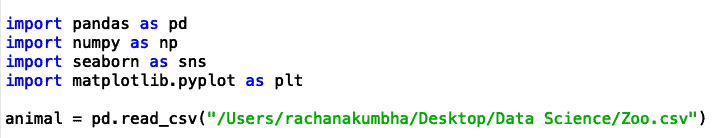Step 2: Analysing the rows and columns will help you understand the dataset.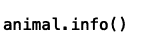There are 18 columns, each with 101 entries, as can be seen. With the exception of the columns with non-numeric values like "animal name" and "type," all of the columns are of the int64 data type. Columns like "hair," "feathers," "eggs," "milk," "airborne," "aquatic," "predator," "tooth," "backbone," "venomous," "domestic," and "catsize" contain categorical binary data; One Hot Encoding was used to convert "Yes" to 1 and "No" to 0.

Using auto EDA tools like pandas_profiling, descriptive statistics and instructive graphical visualisations may be applied to the data.

### Profile Report: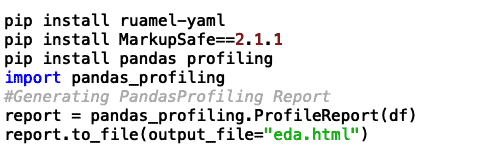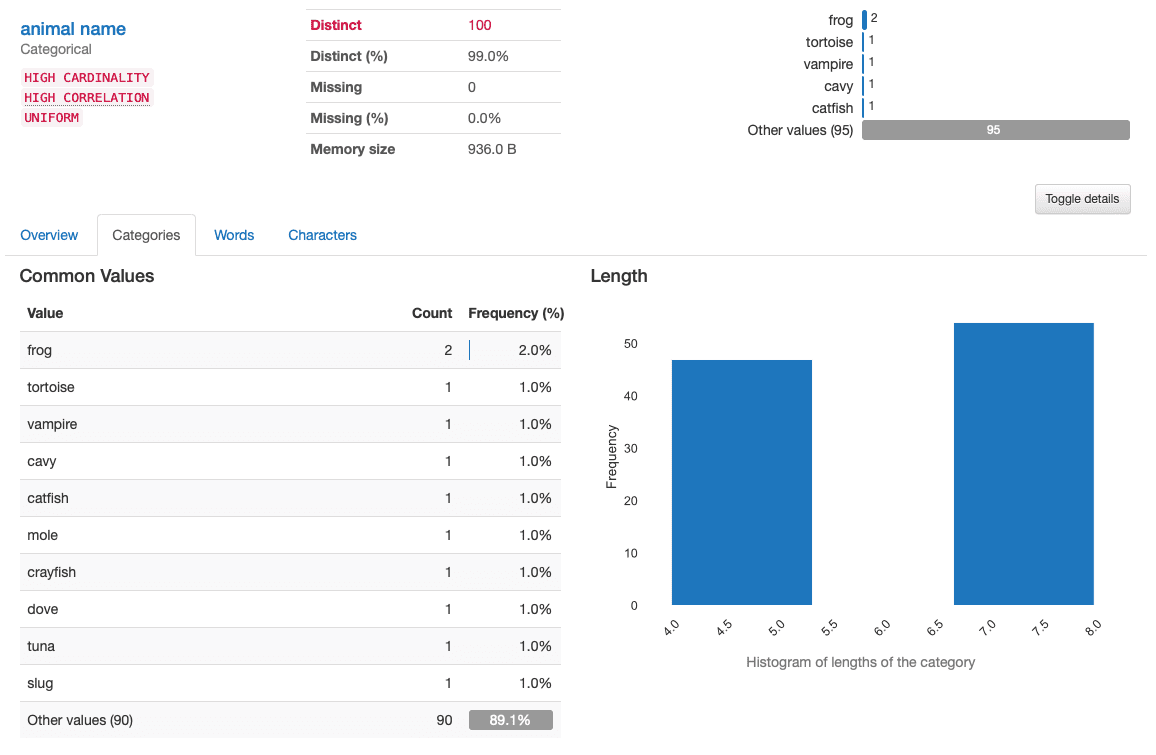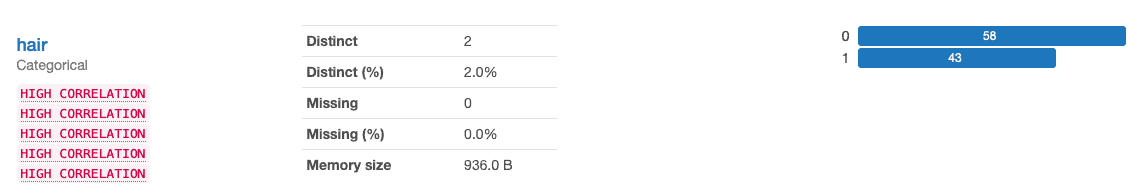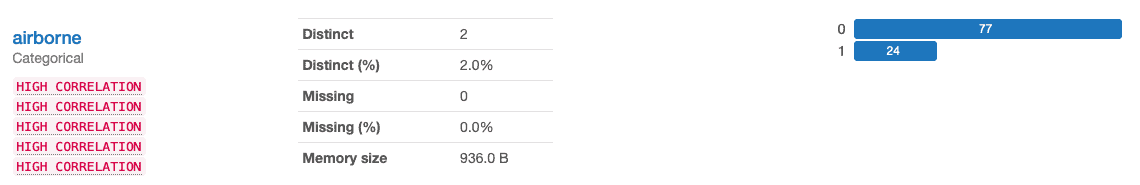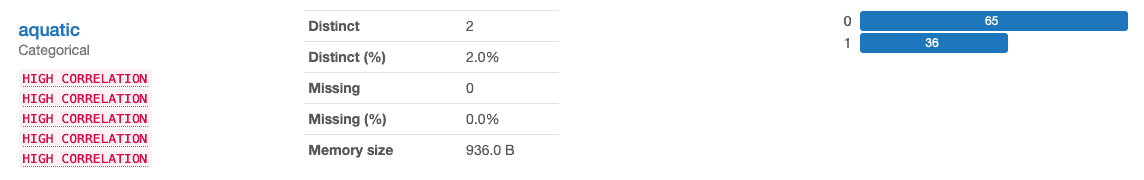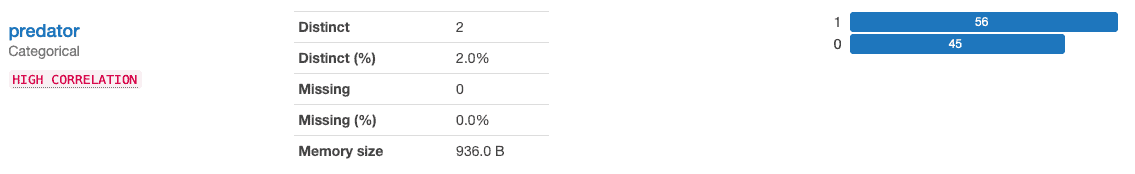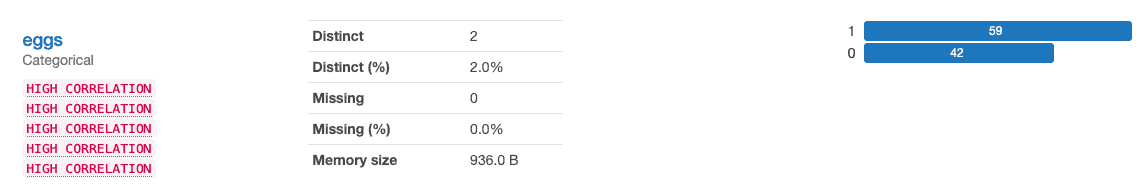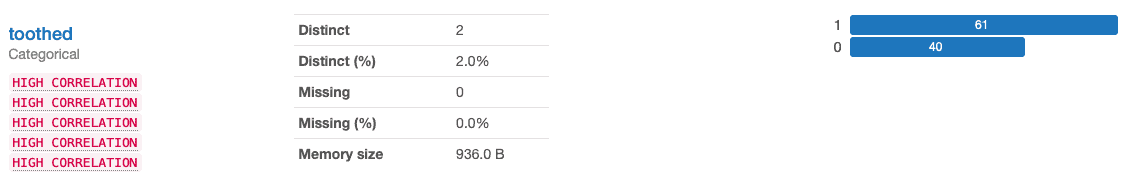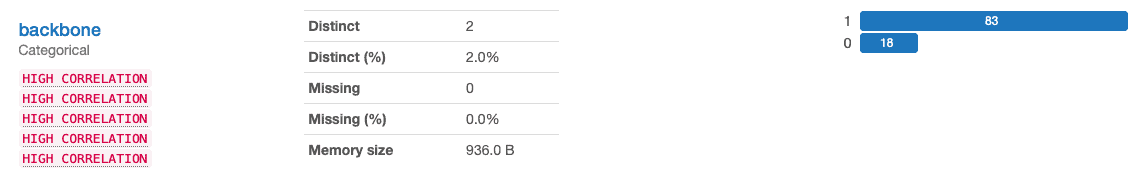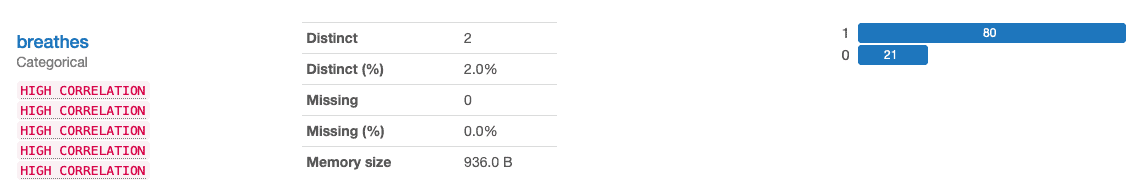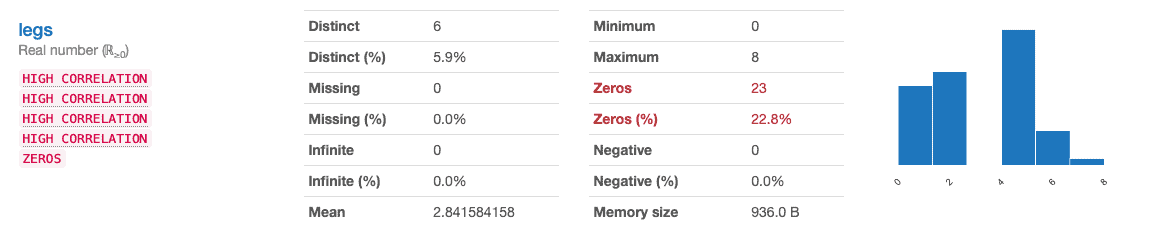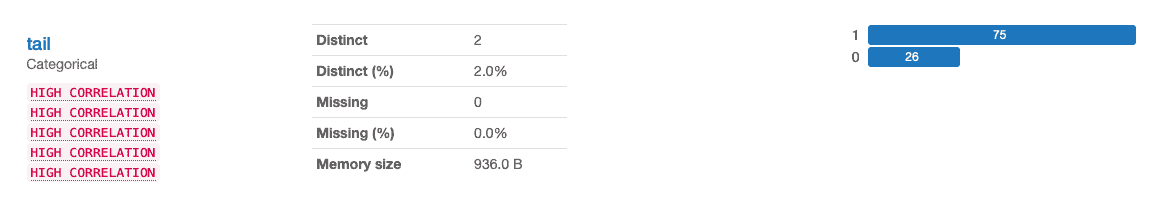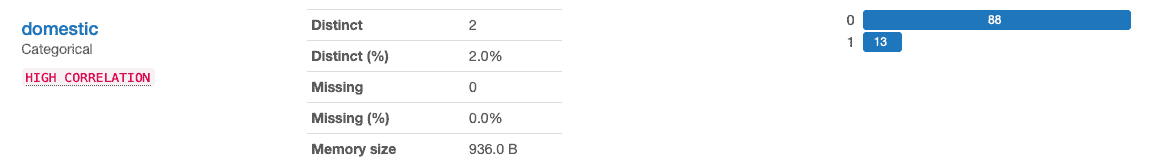We can make the following observations from the above Profile Report:

• We can observe that there are 100 unique animals from the dataset and a total of 101 animals.
• 58 animals are without hair and 43 animals are with hair.
• 81 animals are without feathers and 20 are birds with feathers.
• 77 animals are not airborne and 24 are airborne.
• 65 are non-aquatic and 36 are aquatic animals.
• 56 are not predators and 45 are predators.
• 59 animals do not lay eggs and 42 lay eggs.
• 61 do not have teeth and 40 have teeth.
• 83 do not have a backbone and 18 have a backbone.
• 93 are not venomous and 8 have venom.
• A Maximum number of animals have 4 legs.
• 75 animals have tails and 26 do not have tails.
• 88 are wild animals and 13 are domestic animals.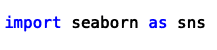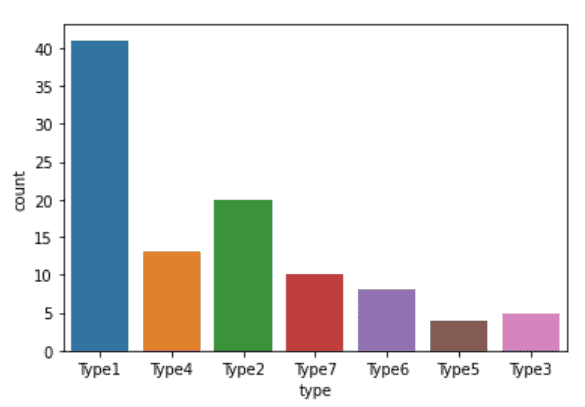We can observe that there are 7 unique types of animals in our dataset where aximum number of animals of Type 1 and least number of animals are of Type 5.

Step 3: We can find the duplicate values and eliminate them as part of the data cleaning process for categorical data. For missing data, mode imputation can also be used.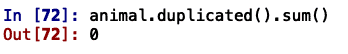Since there are no duplicate values, it is not necessary to eliminate any duplicate rows, as can be seen.Additionally, we see that there are no missing values, therefore no imputation is necessary. We are going to scale all the values to the range of [0,1] using a custom normalisation function since we need to compute the distances between data points and we do not want any characteristic to dominate the distance outcome simply because of large numerical values. We will omit that column from our data normalisation because the "animal name" and "type" columns at indexes 0 and 17 have non-numeric values.Using the describe () method after normalisation, we can determine if the [min, max] numbers have changed to [0, 1].

Step 4: Split the dataset into target and predictor. Further split the target and predictor dataset into train and test data.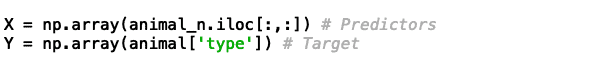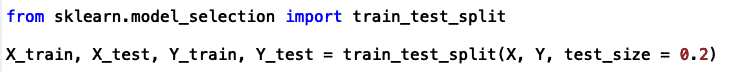Here we are mentioning our test size as 0.2 which means that we are taking 20% of our data for testing and 80% for training.

Step 5: Import KNeighborsClassifier from sklearn module and train our data using the KNeighborsClassifier function with a k (no. of neighbors).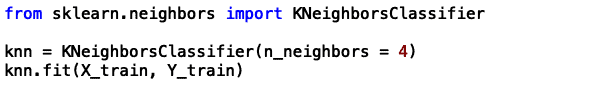Step 6: Predict on our test dataset using the KNN algorithm.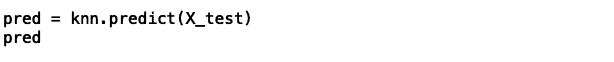Step 7: Using the accuracy score function from the sklearn.metrics module, examine the train and test data for accuracy and error. By utilising the crosstab function to create a confusion matrix, we can also use it to check for true positives, true negatives, false positives, and false negatives for our train and test data.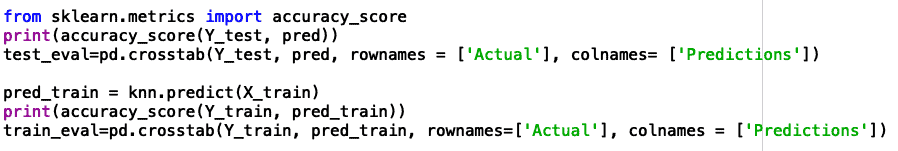Accuracy in tests is 95.2%, while accuracy in training is 93.5%. Its underfitting as a model is understandable. The confusion matrix of the train data shows that one Type 5 animal is expected to be a Type 3 animal. We may conduct more experiments with various k values and select the k value that best fits our model.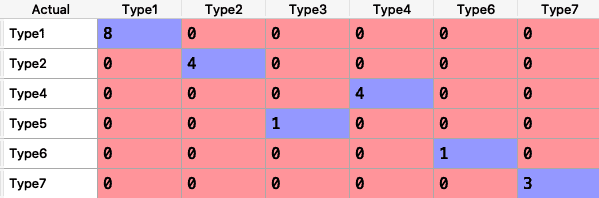Step 8: Generate a classification report for misclassification analysis.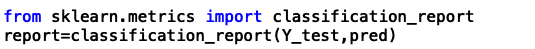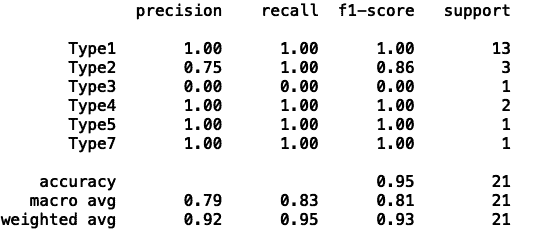Here we can observe that the test accuracy is an aggregate mean of F1-score which is 95%.

Step 9: By experimenting with various values of k using the K-Nearest Neighbours Classifier function and documenting the train and test accuracy for various values of k using the for loop, the optimal value of k may be determined. From k=1 to k=31, we are taking each alternative value.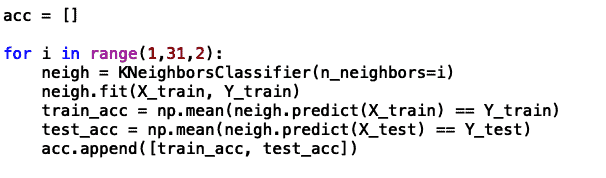[Train accuracy, Test accuracy] is the format used to report accuracy for each value of k. We can see from the data below that, because we are using alternative values, k=3(index=1) provides a correct fit model with train accuracy of 97.5% and test accuracy of 95.2%.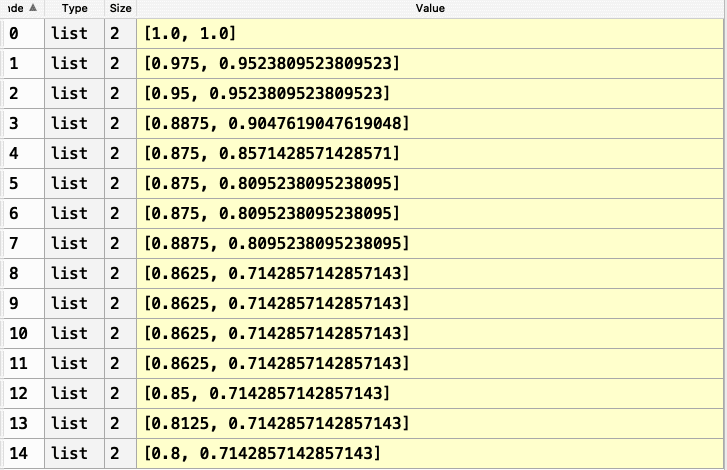Using the code below, we can also see how accurate the train and test are: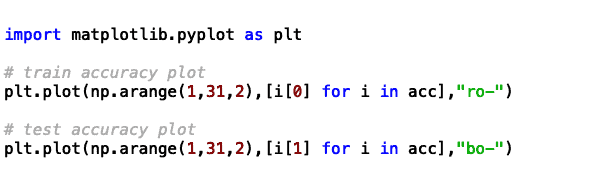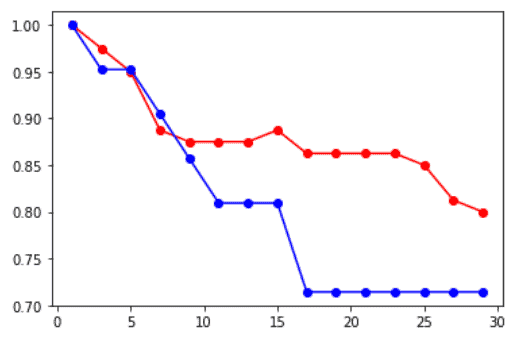We can see that a correct fit model with train accuracy of 97.5% and test accuracy of 95.2% is produced at k=3.

As a result, we may use k=3 to create our final KNN model.

### Advantages of the KNN model:

• It is easy to understand and interpret.
• It is extremely beneficial for non-linear data as the KNN algorithm does not make any underlying assumptions about the data.
• We can use this model for either a numeric target (KNN regressor) or a non-numeric target (KNN classifier).

### Disadvantages of the KNN model:

• In the case of a larger k value, due to numerous distance calculations, we can say that KNN is computationally heavy.
• The KNN model is scaled sensitive even for irrelevant features.
• Memory required is more for KNN as compared to the other models.

### Applications of the KNN model:

• KNN is used in Recommendation Systems of various OTT platforms like Netflix, Prime, YouTube, etc. for customized recommendations.
• KNN is used in Text Mining to identify documents containing similar topics.
• KNN is used in the Banking sector for loan approvals and credit fraud detection.

Click here to learn Data Science Course, Data Science Course in Hyderabad, Data Science Course in Bangalore

### Data Science Training Institutes in Other Locations